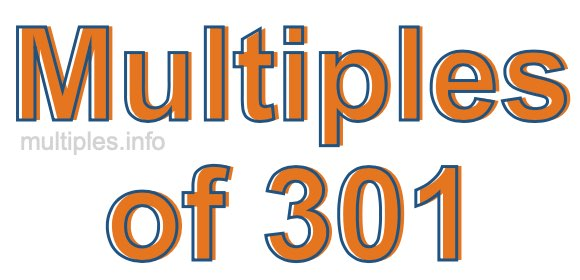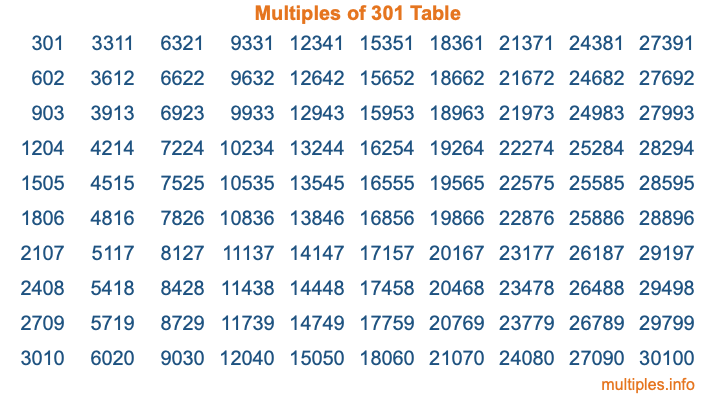Multiples of 301Welcome to the Multiples of 301 page. Here we will first teach you everything you will ever need to know about the multiples of 301, and then give you a study guide summary of everything we taught you to make sure you remember it all. Use this page to look up facts and learn information about the multiples of 301. This page will make you a multiples of three hundred one expert!

Definition of Multiples of 301
Multiples of 301 are all the numbers that when divided by 301 equal an integer. Each of the multiples of 301 are called a multiple. A multiple of 301 is created by multiplying 301 by an integer.

Therefore, to create a list of multiples of 301, you start with 1 multiplied by 301, then 2 multiplied by 301, then 3 multiplied by 301, and so on for as long as you want. Thus, the list of the first five multiples of 301 is 301, 602, 903, 1204, and 1505. To see a larger list of multiples of 301, see the printable image of Multiples of 301 further down on this page. We also have a category where you can choose any nth multiple of 301.

Multiples of 301 Checker
The Multiples of 301 Checker below checks to see if any number of your choice is a multiple of 301. In other words, it checks to see if there is any number (integer) that when multiplied by 301 will equal your number. To do that, we divide your number by 301. If the the quotient is an integer, then your number is a multiple of 301.

Is  a multiple of 301?

Least Common Multiple of 301 and ...
A Least Common Multiple (LCM) is the lowest multiple that two or more numbers have in common. This is also called the smallest common multiple or lowest common multiple and is useful to know when you are adding our subtracting fractions. Enter one or more numbers below (301 is already entered) to find the LCM.

Check out our LCM Calculator if you need more details about the Least Common Multiple or if you need the LCM for different numbers for adding and subtraction fractions.

nth Multiple of 301
As we stated above, 301 is the first multiple of 301, 602 is the second multiple of 301, 903 is the third multiple of 301, and so on. Enter a number below to find the nth multiple of 301.

th multiple of 301

Multiples of 301 vs Factors of 301
301 is a multiple of 301 and a factor of 301, but that is where the similarities end. All postive multiples of 301 are 301 or greater than 301. All positive factors of 301 are 301 or less than 301.

Below is the beginning list of multiples of 301 and the factors of 301 so you can compare:

Multiples of 301: 301, 602, 903, 1204, 1505, etc.

Factors of 301: 1, 7, 43, 301

As you can see, the multiples of 301 are all the numbers that you can divide by 301 to get a whole number. The factors of 301, on the other hand, are all the whole numbers that you can multiply by another whole number to get 301.

It's also interesting to note that if a number (x) is a factor of 301, then 301 will also be a multiple of that number (x).

Multiples of 301 vs Divisors of 301
The divisors of 301 are all the integers that 301 can be divided by evenly. Below is a list of the divisors of 301.

Divisors of 301: 1, 7, 43, 301

The interesting thing to note here is that if you take any multiple of 301 and divide it by a divisor of 301, you will see that the quotient is an integer.

Multiples of 301 Table
Below is an image of the first 100 multiples of 301 in a table. The table is in chronological order, column by column. The first column has the first ten multiples of 301, the second column has the next ten multiples of 301, and so on.The Multiples of 301 Table is also referred to as the 301 Times Table or Times Table of 301. You are welcome to print out our table for your studies.

Negative Multiples of 301
Although not often discussed or needed in math, it is worth mentioning that you can make a list of negative multiples of 301 by multiplying 301 by -1, then by -2, then by -3, and so on, to get the following list of negative multiples of 301:

-301, -602, -903, -1204, -1505, etc.

Multiples of 301 Summary
Below is a summary of important Multiples of 301 facts that we have discussed on this page. To retain the knowledge on this page, we recommend that you read through the summary and explain to yourself or a study partner why they hold true.

There are an infinite number of multiples of 301.

A multiple of 301 divided by 301 will equal a whole number.

301 divided by a factor of 301 equals a divisor of 301.

The nth multiple of 301 is n times 301.

The largest factor of 301 is equal to the first positive multiple of 301.

301 is a multiple of every factor of 301.

301 is a multiple of 301.

A multiple of 301 divided by a divisor of 301 equals an integer.

301 divided by a divisor of 301 equals a factor of 301.

Any integer times 301 will equal a multiple of 301.

Multiples of a Number
Here you can get the multiples of another number, all with the same attention to detail as we did for multiples of 301 on this page.

Multiples of
Multiples of 302
Did you find our page about multiples of three hundred one educational? Do you want more knowledge? Check out the multiples of the next number on our list!